#Function Repository Resource:

# GroupByList

Group elements according to a list of equivalence classes

Contributed by: Carl Woll
 ResourceFunction["GroupByList"][{elem1,elem2,…}, {equiv1, equiv2,…} ] gives an association that groups the elemi into lists associated with distinct keys equivi. ResourceFunction["GroupByList"][{elem1,elem2,…}, {equiv1, equiv2,…} ,red] applies the function red to reduce lists of values that are generated.

## Details and Options

GroupByList[list, equiv] gives an association whose keys are the distinct equivi and whose values are sublists of the list list.
GroupByList[f/@data, g/@data] is equivalent to GroupBy[data, fg].
The association keys equivi are ordered according to their first appearance in the list of equivalence classes.

## Examples

### Basic Examples

Group elements based on their equivalence classes:

 In:=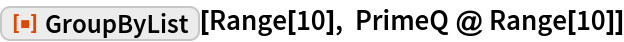Out=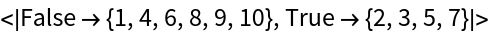### Scope

Consider two datasets:

 In:=Out=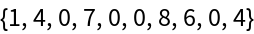In:=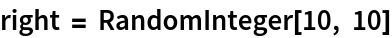Out=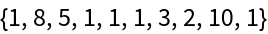Group the positions by the relative order of left and right:

 In:=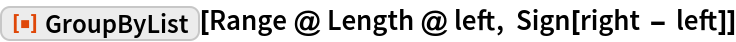Out=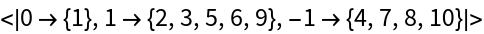### Applications

Find all partitions of the list {a,b,c,d,e} with sublists of length 2, 2 and 1. The possible permutations are:

 In:=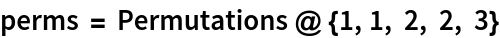Out=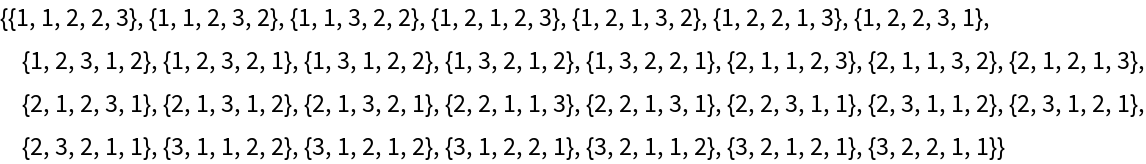Each permutation gives an equivalence class of the list:

 In:=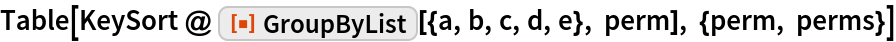Out=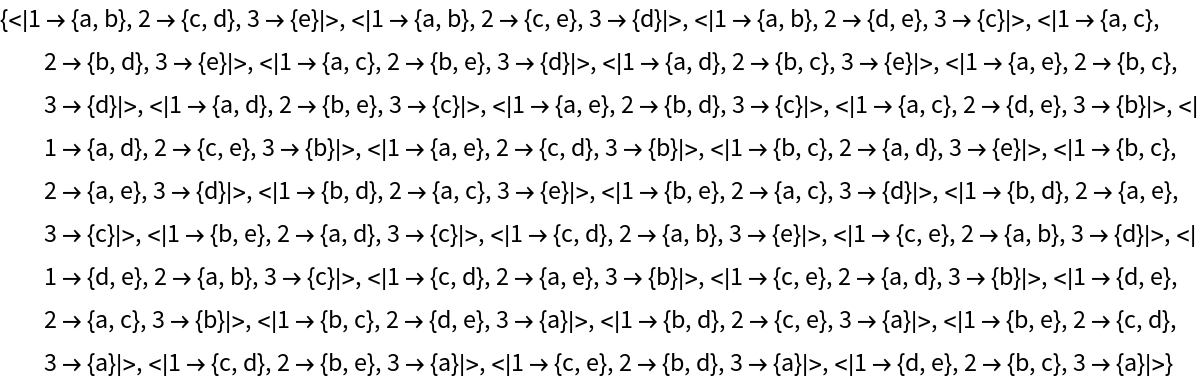### Properties and Relations

GroupByList uses a list of equivalence classes:

 In:=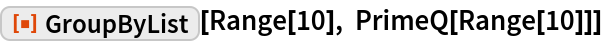Out=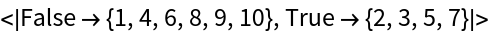GroupBy uses a equivalence function:

 In:=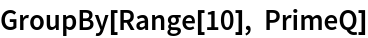Out=GroupByList[f/@data,g/@data] is equivalent to GroupBy[data,fg]. Using GroupByList:

 In:=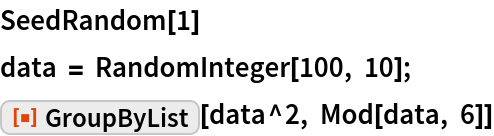Out=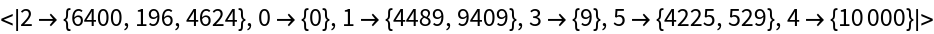Using GroupBy:

 In:=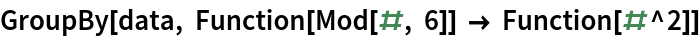Out=